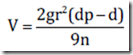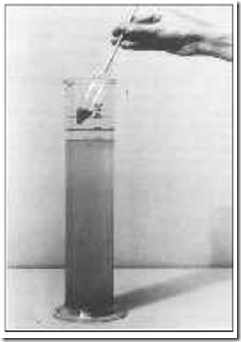Home > English > Soil Texture Determination In Laboratory

Soil Texture Determination In Laboratory

Soil is composed of soil grains of various sizes. Part grain land measuring more than 2 mm is called coarse material soil such as gravel, coral to rock when analyzed eliminated terelebih advance, as well as the organic material in the soil, so all that remains is fractions of land measuring less than 2 mm , Part ground grain size is less than 2 mm called finely ground material.

Finely ground material is divided into:
o Sand (sand), the soil grains measuring between 0,050 mm to 2 mm.
o Dust (silt), the soil grains measuring between 0,002 mm to 0,050 mm.
o Clay (clay), which is the land grain size of less than 0.002 mm.

Determination of texture in the lab can be done by mechanical analysis. The second method is often used to determine the texture, namely, (1) a method pipette and (2) the method hydrometer. In this way the method dropper or method hydrometer, both based on the difference in the speed of the fall of the soil particles in the water with the assumption that the speed of the fall of the particles have a density (density) is the same in a solution will increase linearly when the radius of the particles increases as a quadratic ,

This process consists of dispersing the soil aggregates into single grains and then followed by sedimentation or precipitation. This assumption formulated by Stokes as follows:Where :
v: speed of the fall of the particles (cm / sec)
g: acceleration of gravity (cm / sec)
dp: particle density
d: density of solution (g / cm3)
r: radius of the particles (cm)
n: the absolute viscosity solution (dyne sec / cm2)

Through the method hydrometer soil fractions differentiated by the time the speed falls, which are divided into three groups, namely:
a. Sand fraction are particles that fall to the bottom of the suspension in less than 40 seconds,
b. Fraction dust down between 40 seconds to nearly two hours, and
c. The rest are still suspended the clay fraction.Figure 1.7. Soil Texture Determination Method with hydrometer

The percentage results of the determination of each soil fraction is then matched with the texture triangle, Figure 1.7. Suppose a ground after analysis hydrometer means it contains 50% sand, 20% of dust, and 30% clay. To determine the type of soil texture can be done by comparing the results of data analysis of the three factions land on the image texture triangle. As for how to determine soil texture classes are as follows: 

• From the pictures we can see that the texture triangle on the bottom right corner of the triangle illustrate 0% sand and 100% sand left corner. Locate the point 50% sand on the bottom side of the triangle and from this point draw a line parallel to the right side of the triangle (to the top left).
• Then find the point of 20% of dust on the right side of the triangle. From this point draw a line parallel to the left side of the triangle, so that this line intersects with the first line.
• Then find the point of 30% clay and draw a line to the right parallel to the bottom side of the triangle so that it intersects the two previous lines.

From the intersection of these three lines, it was found that this land has the texture classes sandy clay loam."Tasikmalaya, Indonesia"